# A Deep Learning Algorithm for Solar Radiation Time Series Forecasting: A Case Study of El Kelaa Des Sraghna City

A Deep Learning Algorithm for Solar Radiation Time Series Forecasting: A Case Study of El Kelaa Des Sraghna City

Mohammed Ali JallalAbdessalam El Yassini Samira Chabaa Abdelouhab Zeroual Saida Ibnyaich

I2SP Research Team, Physics Department, Faculty of Sciences Semlalia, Cadi Ayyad University, Marrakesh 40000, Morocco

Industrial Engineering Department, National School of Applied Sciences, Ibn Zohr University, Agadir 80000, Morocco

Corresponding Author Email:
mohammedali.jallal@edu.uca.ac.ma
Page:
563-569
|
DOI:
https://doi.org/10.18280/ria.340505
26 July 2020
|
Accepted:
12 October 2020
|
Published:
20 November 2020
| Citation

OPEN ACCESS

Abstract:

Nowadays, the studies that address solar radiation (SR) forecasting tend to focus on the implementation of conventional techniques. This provides good results, but researchers should focus on the creation of new methodologies that help us in going further and boost the prediction accuracy of SR data. The prime aim of this research study is to propose an efficient deep learning (DL) algorithm that can handle nonlinearities and dynamic behaviors of the meteorological data, and generate accurate real-time forecasting of hourly global solar radiation (GSR) data of the city of El Kelaa des Sraghna (32°2’53”N 7°24’30”W), Morocco. The proposed DL algorithm integrates the dynamic model named Elman neural network with a new input configuration-based autoregressive process in order to learn from the seasonal patterns of the historical SR measurements, and the actual measurements of air temperature. The attained performance proves the reliability and the accuracy of the proposed model to forecast the hourly GSR time series in case of missing values detection or pyranometer damage. Hence, electrical power engineers can adopt this forecasting tool to improve the integration of solar power resources into the power grid system.

Keywords:

artificial intelligence, global solar radiation, deep learning, Elman neural network, forecasting, time series

1. Introduction

The power companies and decision-makers are still facing the challenging task of incorporating renewable power shares into electrical grids. Green power technology is a promising alternative for electricity production, particularly solar photovoltaic (PV) technology, since it is a clean source of electricity .

In the last years, by realizing the benefits of PV technology, large PV solar plants have been implemented worldwide. In order to commercialize and use this technology on a large scale, several issues have to be resolved.

Most of the challenging issues to boost the integration of solar power production into the electrical grid are its dependency on weather conditions. Hence, an accurate prediction of solar radiation measurements is a mandatory step for controlling, sizing, and designing PV systems.

Solar radiation estimation has been extensively considered by implementing various estimating approaches. Statistical techniques-based time series regression  and data driven-based artificial intelligence (AI) algorithms  are two commonly employed estimating tools. Among the most suitable techniques implemented to SR time series forecasts are AI techniques since these techniques do not necessitate a clear explanation of the sun's physical characteristics to carry out this type of prediction.

The artificial neural networks (ANNs) have experienced a vital evolution during the last years and is extensively employed in different engineering areas, such as the green power sector [4, 5], medicine [6, 7], civil engineering , computer vision [9, 10], and so on.

According to our literature review, several researches based on ANNs and other machine learning algorithms have been conducted to perform accurate solar radiation forecasts.

Jallal et al. proposed a new ensemble technique based on artificial neural network to estimate the hourly global solar radiation time series, where the reliability is evaluated using 7 years of measurement of several meteorological data . The same authors develop an artificial neuron combined with an autoregressive process to predict GSR parameter. The developed model performed accurate predictions during 6 years, which is reserved for the testing process .

Kisi et al. applied a dynamic neuro-fuzzy inference forecasting approach based on mono-variate AT structure to estimate GSR data .

Mousavi et al. have proposed a new training strategy based on simulated annealing optimizer to train the ANN’s parameters in order to generate precise daily GSR predictions .

Xue et al. compare the training performance of genetic and particle swarm optimization (PSO) algorithms to tune ANN’s weights and biases for forecasting accurately daily GSR measurements. The authors demonstrate the efficiency of adopting PSO optimizer than the genetic algorithm .

Garcia-Hinde et al. developed a smart learning approach based on reducing the dimensionality curse in the input layer for generating precise solar radiation time series estimation .

In the reviewed studies for SR time series prediction, we have faced the following issues:

• The temporal features of solar radiation time series have not been considered by most of the reviewed papers,
• The development of a forecasting model to infer the correlation existing between the input and the output patterns in an effective way without adopting any features selection or normalization techniques is not frequently treated or enhanced,
• Most of the proposed approaches have a static configuration, where dynamic models are required to address the non-linear behavior of SR measurements, as Elman neural network technique.

Motivated by the above mentioned researches, the prime keys of this article are:

• Proposing an accurate forecasting tool for power engineers based on a deep learning algorithm to improve renewable energy resources integration into the power grid,
• Adopting a dynamic neural network, named Elman neural network (ENN) to approximate dynamic and strong nonlinearity of solar radiation time series, which depend on the chaotic behavior of weather conditions,
• The developed technique forecasts successfully the hourly global solar radiation data of El Kelaa des Sraghna city with high accuracy. Hence, the developed model can be used to generate synthetic data of GSR in case of missing values detection or pyranometer damage.

The remaining of this article is written as follows: In section 2, the adopted dataset of the city of El Kelaa Des Sraghna and the theory behind the Elman neural network are presented. In Section 3, the forecasting results of the proposed approach are presented and discussed. Finally, in Section 4 conclusions, are given.

2. Dataset and Methods

2.1 El Kelaa Des Sraghna’s dataset description

In this research study, the typical hourly year of global solar radiation (GSR) and air temperature (AT) measurements-based Meteonorm software are adopted to train and evaluate the proposed forecasting DL algorithm.

A commonly debated problem related to the correlation models to forecast SR data is the appropriate selection of the input variables. A detailed review is discussed by Yadav et al. , which confirms the high correlation of the endogenous SR’s variables and the exogenous AT measurements as inputs.

## 1.png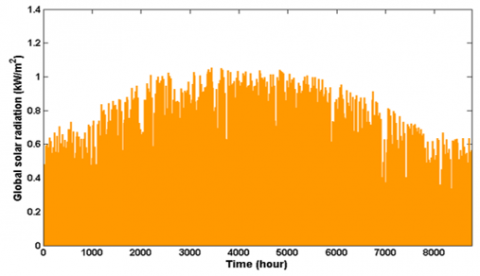Figure 1. Global solar radiation time series evolution

## 2.png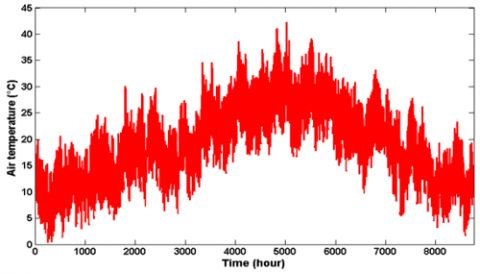Figure 2. Air temperature time series evolution

The adopted GSR and AT measurements are recorded on the hourly scale in the city of El Kelaa des Sraghna, Morocco. This city is located in the center of Morocco, and the city's economy depends on farming, with the primary growth of olive trees.

Based on the Koppen climat classification, the city has a hot semi-arid climate (BSh).

In Figures 1 and 2, the GSR and AT time series are presented, respectively.

2.2 Theory behind Elman neural network

A multi-layer artificial neural network's standard architecture consists of three layers that encompass artificial neurons, including the input layer, the hidden layer, and the output layer . The input layer nodes’ number matches the system input’s data dimension. All of the input signals are affected with synaptic weights and then transmitted to the hidden layer that is characterized by a specific activation function. Then, the signals of the output layer’s neurons are computed based on the weighted hidden layer’s outputs .

However, solar radiation (SR) forecasting is a complex task, which is due to strong nonlinear and dynamic behaviors of SR time series [12, 19]. In order to address these issues, the past output data of the developed predictive model can be involved in the input’s data vector by adopting an external time delay operator if the typical feedforward ANN technique is applied . This raises the input’s data vector dimension, increases computational time, and reduces convergence speed .

Thus, a recurrent ANN model is required. An ENN model is adopted in the present paper. In 1990, The ENN was developed by Jeffrey L. Elman, which is a recurrent ANN technique that has super computational power .

As Figure 3 demonstrated, it encompasses four layers, namely the input layer, the hidden layer, the context links layer, and the output layer [21, 22].

Compared to feedforward ANNs such as the backpropagation ANN , there is an additional layer named the context links layer that receives feedback signals from the hidden layer and store them as historical data. Then, these historical data are used as inputs for the hidden layer via the context links layer that is associated with a delay operator $Z^{-T D L}$ of order TDL .

The context links layer boost the learning ability of ANN technique to approximate dynamic and nonlinear processes, such as solar radiation time series forecasting .

The ENN model is supposed to have d inputs’ features $\left\{x_{1}, x_{2}, \ldots, x_{d}\right\}$, h neurons in the hidden layer $\left\{H_{1}, H_{2}, \ldots, H_{h}\right\}$, a context links layer $\left\{C_{1}, C_{2}, \ldots, C_{m}\right\}$ with m dimension, and n outputs’ signals $\left\{y_{1}, y_{2}, \ldots, y_{n}\right\}$ [19, 20, 22].

## 3.pngFigure 3. Typical architecture of Elman neural network

The output signal of the kth context link is defined as:

$C_{k}(t)=H_{k}(t-1)$    (1)

where, t and t-1 represents, the actual time and the previous time step, respectively. The weighted input signals Ak of the kth hidden neuron are computed as follow:

$A_{k}(t)=\sum_{i=1}^{d} \omega_{i k} x_{i}(k)+\theta_{k}$   (2)

where,

ωik are the synaptic weights between the input layer and the kth hidden neuron, θk is the bias of the kth neuron.

The output signal of the kth hidden neuron is calculated by the following equation:

$H_{k}(t)=\Psi\left(A_{k}(k)+W^{*} C_{k}(t-1)\right)$    (3)

where,

Ψ is the activation function of the hidden layer’s neuron,

W* are the weights of the context links layer.

The ENN jth output are computed by Eq. (4):

$y_{j}(t)=\Phi\left(\sum_{l=1}^{h} \omega_{l j} H_{l}(t)\right)$   (4)

where,

ωlj are the weights between the hidden layer and the output layer, Φ is the output layer activation function. Several activation functions can be adopted by the hidden neurons [11, 24], such as:

Logistic sigmoid function:

$f(x)=\frac{1}{1+e^{-x}}$    (5)

Hyperbolic tangent sigmoid function:

$f(x)=\frac{e^{x}-e^{-x}}{e^{x}+e^{-x}}$   (6)

$f(x)=\exp \left(-\frac{1}{\sigma^{2}}\|x-\mu\|^{2}\right)$      (7)

Linear function:

$f(x)=x$     (8)

where, $\sigma^{2}$ is the variance and μ is the center or (mean).

ENN model’s parameters are tuned using an efficient learning optimizer, which is based on the ENN output’s error minimization that is computed by Eq. (9).

$E=\sum_{i=1}^{N}\left(T_{i}-\hat{y}_{i}\right)^{2}$     (9)

where,

Ti is the ith element of the output target,

$\hat{y}$ is the ith element of the estimated output,

N is the output signal dimention.

In this paper, the Levenberg-Marquardt (LM) optimizer was adopted to tune the ENN model’s parameters .

Based on several pieces of literature, LM optimizer has demonstrated a high efficiency to train several ANN architectures, which are adopted to resolve a myriad of complex tasks. Such as, image processing, antenna design, cybersecurity, meteorological prediction, tracking renewable energy production, and so on.

LM optimizer incorporates both gradient descent and Gauss-Newton techniques in order to boost the estimation stability and the convergence speed of these traditional techniques. This combination can ensure an efficient solution for complex tasks via its adaptive feature .

If the gradient descent technique was adopted, the convergence speed becomes slower, and reaching the optimal solution is not guaranteed . Furthermore, if the Gauss-Newton technique is adopted, there is a high probability of obtaining an optimal solution .

In LM optimizer, the computing of the Hessian matrix is given by Eq. (10), and its gradient computing is defined by Eq. (11):

$H=J^{T} J$    (10)

$G=J^{T} E$     (11)

where, j define the Jacobian matrix and E represent the error calculation given by Eq. (9). Then, the LM optimizer performs as the following updating formula:

$w(t+1)=w(t)-\left[J^{T} J+\rho I\right]^{-1} J^{T} E$    (12)

where,

w(t+1) is the updated weight value,

w(t) is the actual weight value,

ρ is the learning rate coefficient,

I is the identity matrix.

3. Forecasting Results and Discussion

3.1 Proposed forecasting scheme

In this article, ENN technique has been applied to forecast solar radiation measurements of the city of El Kelaa des Sraghna (32°2’53”N 7°24’30”W), Morocco. The developed forecasting model is incorporated an autoregressive process of order 12 AR(12) to the input layer to learn from the seasonal patterns of the historical SR measurements that are recorded during the past 12 hours, and the actual measurements of air temperature.

## 4.png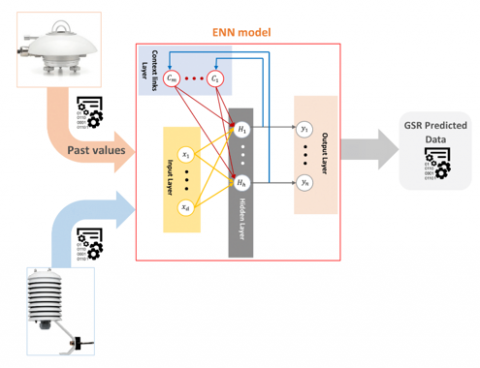Figure 4. Architecture of the proposed forecasting model

## 5.png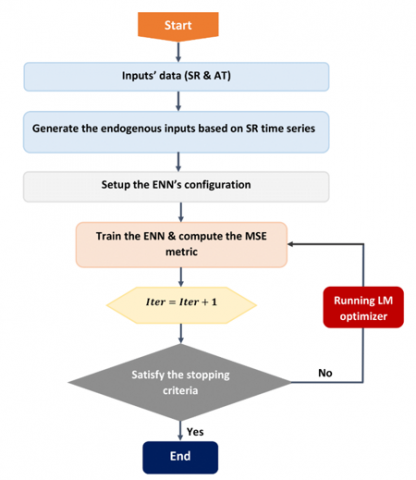Figure 5. Flowchart of the proposed model

The developed predictive model allows the power grid operator to overcome the issues of missing values and generate synthetic data of global solar radiation parameter in case of pyranometer damage or missing values detection.

In Figure 4, the proposed model’s scheme is presented. The proposed forecasting model is trained using the LM optimizer, where 70% of the whole database is used for adjusting the model’s parameters (training process), and the remaining 30% of the database is used to evaluate the reliability and the accuracy of the developed model (testing process).

In Figure 5, the different steps to develop the proposed forecasting model-based ENN technique are presented.

Since there is no efficient strategy to define the optimal hyper-parameters of the proposed learning algorithm, a trial-and-error analysis was conducted to define the optimal number of hidden layers, the number of hidden neurons, and the activation function type based on the minimal value of the mean square error (MSE).

To evaluate the proposed model’s performance, two indicators are used, the mean square error (MSE) and the correlation coefficient (R), which are defined by the following equations :

$M S E=\frac{1}{N} \sum_{i=1}^{N}\left(d_{i}-p_{i}\right)^{2}$    (13)

$R=\sqrt{\sum_{i=1}^{N}\left(p_{i}-d_{m i}\right)^{2} / \sum_{i=1}^{N}\left(d_{i}-d_{m i}\right)^{2}}$     (14)

where,

pi is the estimated output,

Yi is the target,

dmi is the mean value of di,

N is the samples’ size.

The variation of the hidden layer’s number is depicted as function of the MSE metric in Figure 6. The obtained results demonstrate that the adequate ENN structure is the one with a single hidden layer.

## 6.png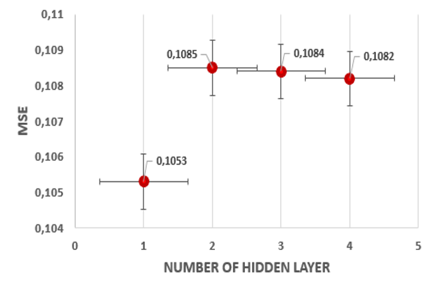Figure 6. Hidden layers number variation versus the MSE metric calculations

## 7.pngFigure 7. Neurons number variation versus the MSE metric calculations

Figure 7 shows the evolution of the hidden neurons’ number versus the MSE values. Based on this variation, it is clear that the adoption of 8 hidden neurons is the optimal option.

For the optimal hidden layer’s activation function, the radial basis function recorded the minimal value of the MSE indicator among other activation functions, such as, purline, sigmoid, and hyper tangent sigmoid function.

From the attained results, which is depicted in Figure 8, the best context links order TDL is 9.

## 8.png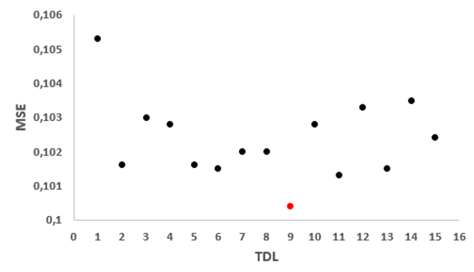Figure 8. Context links order TDL variation versus the MSE metric calculations

3.2 Performance discussion of the developed model

Once the optimum ENN’s structure is defined. The 30% of the remaining dataset that is reserved for performing the testing phase is applied to prove the reliability and the accuracy of the developed forecasting model.

In Figure 9 the SR time series patterns are depicted against the forecasting results’ patterns to evaluate the coexisting agreement between the measured and predicted time series. Based on the achieved results, it is demonstrated that there a high relationship between the measured and predicted time series during the testing process.

## 9.png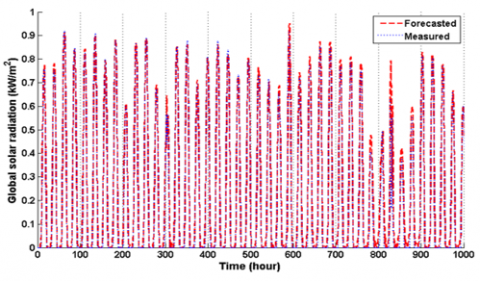Figure 9. Forecasted versus measured global solar radiation patterns during the testing process

To reinforce the evaluation process, a scattering graph of measured and predicted global solar radiation (GSR) data is presented in Figure 10. The attained results show a high correlation between real and forecasted GSR measurements.

The attained accuracy in Figures 9 and 10 demonstrates the proposed approach's efficiency to be applied by power grid operators and engineers to boost renewable power capacity integration into the power grid.

From the results presented in Table 1, the proposed forecasting approach attained a higher SR prediction precision compared to diverse estimating approaches introduced in different pieces of the literature in terms of the correlation coefficient metric.

## 10.png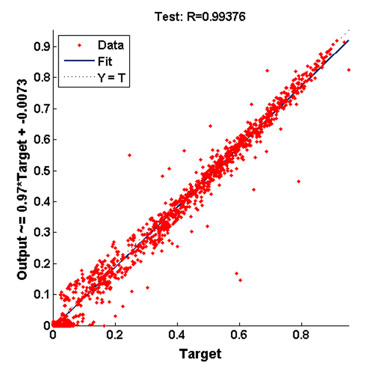Figure 10. Scattering graph of forecasted and real global solar radiation data during the testing process

Table 1. Comparison of the proposed technique with different statistical and DL-based methods for predicting solar radiation time series

 References Locations Applied methods R (%)  Beijing, China Empirical model 93  90 stations, China ResnetTL 88  Hong Kong, China MARS 91.3  Al Ain, UAE ANN 92  Adana, Turkey DENFIS 94.2  Antakya, Turkey DENFIS 97.9  China ANFIS-SC 81-94  China ANFIS-GP 82-91  China M5Tree 71-86 Present study Marrakesh, Morocco Proposed approach 99.38
4. Conclusions

Recently, the demand for solar energy recognized a significant increase. Therefore, solar power engineers need to implement sophisticated tools-based AI to boost the integration of solar energy into the electric grid utility. In this research study, a dynamic deep learning algorithm based on Elman neural network was proposed to forecast the hourly global solar radiation time series of the city of El Kelaa des Sraghna, Morocco. The forecasting results were performed using historical SR measurements measured over the last 12 hours, as endogenous inputs, and actual air temperature data as exogenous inputs. The achieved value of the R metric that is recorded during the testing phase is about 99.38%. This attained correlation’s value proves the proposed learning algorithm’s effectiveness and its reliability to be adopted by power grid engineers in case of missing values detection or solar data acquisition chain damage.

References

 Phuangpornpitak, N., Tia, S. (2013). Opportunities and challenges of integrating renewable energy in smart grid system. Energy Procedia, 34: 282-290. https://doi.org/10.1016/j.egypro.2013.06.756

 Besharat, F., Dehghan, A.A., Faghih, A.R. (2013). Empirical models for estimating global solar radiation: A review and case study. Renewable and Sustainable Energy Reviews, 21: 798-821. https://doi.org/10.1016/j.rser.2012.12.043

 Voyant, C., Notton, G., Kalogirou, S., Nivet, M.L., Paoli, C., Motte, F., Fouilloy, A. (2017). Machine learning methods for solar radiation forecasting: A review. Renewable Energy, 105: 569-582. https://doi.org/10.1016/j.renene.2016.12.095

 Jallal, M.A., Chabaa, S., Zeroual, A. (2020). A novel deep neural network based on randomly occurring distributed delayed PSO algorithm for monitoring the energy produced by four dual-axis solar trackers. Renewable Energy, 149: 1182-1196. https://doi.org/10.1016/j.renene.2019.10.117

 Mouachi, R., Jallal, M.A., Gharnati, F., Raoufi, M. (2020). Multiobjective sizing of an autonomous hybrid microgrid using a multimodal delayed PSO algorithm: A case study of a fishing village. Computational Intelligence and Neuroscience, 2020: 1-18. https://doi.org/10.1155/2020/8894094

 Lisboa, P.J. (2002). A review of evidence of health benefit from artificial neural networks in medical intervention. Neural Networks, 15(1): 11-39. https://doi.org/10.1016/S0893-6080(01)00111-3

 Schwarzer, G., Vach, W., Schumacher, M. (2000). On the misuses of artificial neural networks for prognostic and diagnostic classification in oncology. Statistics in Medicine, 19(4): 541-561. https://doi.org/10.1002/(SICI)1097-0258(20000229)19:4<541::AID-SIM355>3.0.CO;2-V

 Flood, I., Kartam, N. (1994). Neural networks in civil engineering. I: Principles and understanding. Journal of computing in Civil Engineering, 8(2): 131-148. https://doi.org/10.1061/(asce)0887-3801(1994)8:2(131)

 Bishop, C.M. (2007). Pattern recognition and machine learning. Journal of Electronic Imaging, 16(4): 049901. https://doi.org/10.1117/1.2819119

 Tran, D., Bourdev, L., Fergus, R., Torresani, L., Paluri, M. (2015). Learning spatiotemporal features with 3d convolutional networks. 2015 IEEE International Conference on Computer Vision (ICCV), Santiago, pp. 4489-4497. https://doi.org/10.1109/ICCV.2015.510

 Jallal, M.A., Chabaa, S., Zeroual, A. (2020). A new artificial multi-neural approach to estimate the hourly global solar radiation in a semi-arid climate site. Theoretical and Applied Climatology, 139(3-4): 1261-1276. https://doi.org/10.1007/s00704-019-03033-1

 Jallal, M.A., El Yassini, A., Chabaa, S., Zeroual, A., Ibnyaich, S. (2020). AI data driven approach-based endogenous inputs for global solar radiation forecasting ai data driven approach-based endogenous inputs for global solar radiation forecasting. Ingénierie des Systèmes d Information, 25(1): 27-34. https://doi.org/10.18280/isi.250104

 Kisi, O., Heddam, S., Yaseen, Z.M. (2019). The implementation of univariable scheme-based air temperature for solar radiation prediction: New development of dynamic evolving neural-fuzzy inference system model. Applied Energy, 241: 184-195. https://doi.org/10.1016/j.apenergy.2019.03.089

 Mousavi, S.M., Mostafavi, E.S., Jiao, P. (2017). Next generation prediction model for daily solar radiation on horizontal surface using a hybrid neural network and simulated annealing method. Energy Conversion and Management, 153: 671-682. https://doi.org/10.1016/j.enconman.2017.09.040

 Xue, X. (2017). Prediction of daily diffuse solar radiation using artificial neural networks. International Journal of Hydrogen Energy, 42(47): 28214-28221. https://doi.org/10.1016/j.ijhydene.2017.09.150

 García-Hinde, O., Terrén-Serrano, G., Hombrados-Herrera, M.A., Gómez-Verdejo, V., Jiménez-Fernández, S., Casanova-Mateo, C., Salcedo-Sanz, S. (2018). Evaluation of dimensionality reduction methods applied to numerical weather models for solar radiation forecasting. Engineering Applications of Artificial Intelligence, 69: 157-167. https://doi.org/10.1016/j.engappai.2017.12.003

 Yadav, A.K., Mali, H., Chandel, S.S.  (2014). Selection of most relevant input parameters using WEKA for artificial neural network based solar radiation prediction models. Renewable Sustainable Energy Reviews, 31: 509-519. https://doi.org/10.1016/j.rser.2013.12.008

 Jallal, M.A., Chabaa, S., El Yassini, A., Zeroual, A., Ibnyaich, S. (2019). Air temperature forecasting using artificial neural networks with delayed exogenous input. 2019 International Conference on Wireless Technologies, Embedded and Intelligent Systems (WITS), Fez, Morocco, pp. 1-6. https://doi.org/10.1109/WITS.2019.8723699

 Jallal, M.A., Chabaa, S., El Yassini, A., Zeroual, A., Ibnyaich, S., Raoufi, M. (2019). Elman neural network for solar radiation components forecasting based on the desired tilt angle. In 2019 7th International Renewable and Sustainable Energy Conference (IRSEC), Agadir, Morocco, pp. 1-5. https://doi.org/10.1109/IRSEC48032.2019.9078281

 Sun, L., Yi, W., Yuan, D., Guan, J. (2019). Application of Elman neural network based on genetic algorithm in initial alignment of SINS for guided projectile. Mathematical Problems in Engineering, 2019: 1-9. https://doi.org/10.1155/2019/5810174

 Elman, J.L. (1990). Finding structure in time. Cognitive Science, 14(2): 179-211. https://doi.org/https://doi.org/10.1016/0364-0213(90)90002-E

 Pi, J., Huang, J., Ma, L. (2017). Aeroengine fault diagnosis using optimized Elman neural network. Mathematical Problems in Engineering, 2017: 1-8. https://doi.org/https://doi.org/10.1155/2017/9726529

 Schmidhuber, J. (2015). Deep learning in neural networks: An overview. Neural Networks, 61: 85-117. https://doi.org/10.1016/j.neunet.2014.09.003

 Dadkhah, M., Rezaee, M.J., Chavoshi, A.Z. (2018). Short-term power output forecasting of hourly operation in power plant based on climate factors and effects of wind direction and wind speed. Energy, 148: 775-788. https://doi.org/10.1016/j.energy.2018.01.163

 Burney, S., Jilani, T., Ardil, C. (2008). Levenberg-Marquardt algorithm for Karachi stock exchange share rates forecasting. International Journal of Computer and Information Engineering, 2(4): 1330-1335. https://doi.org/10.5281/zenodo.1071284

 Du, Y.C., Stephanus, A. (2018). Algorithm for degree of arteriovenous fistula stenosis classification using a dual optical photoplethysmography sensor. Sensors, 18(7): 2322. https://doi.org/10.3390/s18072322

 Jallal, M.A., González-Vidal, A., Skarmeta, A.F., Chabaa, S., Zeroual, A. (2020). A hybrid neuro-fuzzy inference system-based algorithm for time series forecasting applied to energy consumption prediction. Applied Energy, 268: 114977. https://doi.org/10.1016/j.apenergy.2020.114977

 Chang, K., Zhang, Q. (2019). Improvement of the hourly global solar model and solar radiation for air-conditioning design in China. Renewable Energy, 138: 1232-1238. https://doi.org/10.1016/j.renene.2019.02.069

 Jiang, H., Lu, N., Qin, J., Tang, W., Yao, L. (2019). A deep learning algorithm to estimate hourly global solar radiation from geostationary satellite data. Renewable and Sustainable Energy Reviews, 114: 109327. https://doi.org/10.1016/j.rser.2019.109327

 Li, D.H., Chen, W., Li, S., Lou, S. (2019). Estimation of hourly global solar radiation using Multivariate Adaptive Regression Spline (MARS)–A case study of Hong Kong. Energy, 186: 115857. https://doi.org/10.1016/j.energy.2019.115857

 Al-Shamisi, M.H., Assi, A.H., Hejase, H.A. (2013). Artificial neural networks for predicting global solar radiation in Al Ain city-UAE. International Journal of Green Energy, 10(5): 443-456. https://doi.org/10.1080/15435075.2011.641187

 Wang, L., Kisi, O., Zounemat‐Kermani, M., Zhu, Z., Gong, W., Niu, Z., Liu, Z. (2017). Prediction of solar radiation in China using different adaptive neuro‐fuzzy methods and M5 model tree. International Journal of Climatology, 37(3): 1141-1155. https://doi.org/10.1002/joc.4762